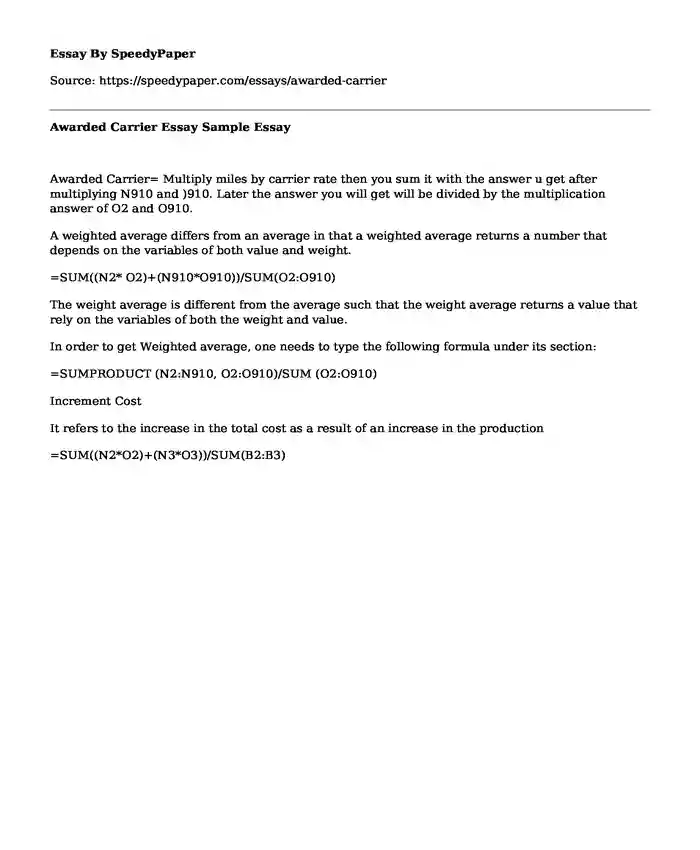# Awarded Carrier Essay Sample

Published: 2017-12-07Type of paper: Essay Categories: Finance Problem solving Pages: 1 Wordcount: 134 words
143 views

Awarded Carrier= Multiply miles by carrier rate then you sum it with the answer u get after multiplying N910 and )910. Later the answer you will get will be divided by the multiplication answer of O2 and O910.

Is your time best spent reading someone else’s essay? Get a 100% original essay FROM A CERTIFIED WRITER!

A weighted average differs from an average in that a weighted average returns a number that depends on the variables of both value and weight.

=SUM((N2* O2)+(N910*O910))/SUM(O2:O910)

The weight average is different from the average such that the weight average returns a value that rely on the variables of both the weight and value.

In order to get Weighted average, one needs to type the following formula under its section:

=SUMPRODUCT (N2:N910, O2:O910)/SUM (O2:O910)

Increment Cost

It refers to the increase in the total cost as a result of an increase in the production

=SUM((N2*O2)+(N3*O3))/SUM(B2:B3)